# Problems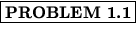What is the net force (magnitude and direction) on the electron moving in the magnetic field in Fig. 1.10 if B = 2 T, v = 4 x 104 m/s, and= 30 o ?Solution:
We first find the magnitude of the force as

F = qvBsin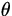= 1.6 x 10- 194 x 1042sin 30 o = 6.4 x 10- 15 N .

To determine the direction, we note thatx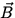is directed out of the page, and so the force on a negative charge is opposite to this. Thus, the force on the electron is 6.4 x 10- 15 N directed into the page.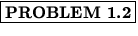Suppose a very long straight wire of linear mass density 20 g/m is immersed in a constant magnetic field B = 3 T, as in Fig. 1.11. What current I would be required so that the wire will be suspended?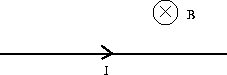Solution:
We note that the force per unit length due to gravity,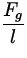=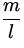g,

is directed downwards, while the force per unit length due to the magnetic field,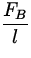= IB,

is acting upwards. In order that they balance, we demandg = IB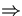I =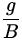=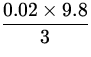= 0.065 A .

Thus, the wire must carry a current of 0.065 A in order to be suspended.Consider the mass spectrometer in Fig. 1.12. The electric field between the plates of the velocity selector is E = 950 V/m, and the magnetic field B in both the velocity selector and in the deflection chamber has a magnitude of 0.9 T. Find the radius r for a singly charged ion of mass m = 2.18 x 10- 26 kg in the deflection chamber.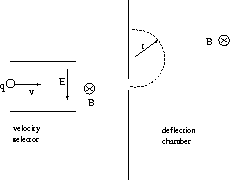Solution:
We first use the fact that in order for the ion to pass through the velocity selector undeflected the force due to the electric and magnetic fields must balance. Note that the force on a positive charge is downwards due to the electric field and upwards due to the magnetic field; to balance, we then have

qE = qvBv =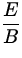.

When the ion enters the deflection chamber it experiences a magnetic force, causing it to go in a circular orbit. The magnetic force then gives rise to the centripetal acceleration as

F = qvB = m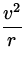qB =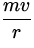.

We thus find

r =v =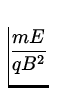== 1.6 x 10- 4 m .

Thus, the radius of the orbit is 0.16 mm.The two long straight wires in Fig. 1.13 each carry a current of I = 5 A in opposite directions and are separated by a distance d = 30 cm. Find the magnetic field a distance l = 20 cm to the right of the wire on the right.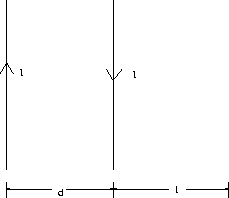Solution:
We shall use the expression for the magnetic field of a long straight wire a distance r away as

B =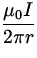,

with direction given by the appropriate right-hand-rule. Due to the wire on the right, the magnetic field is

BR =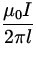=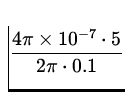= 1.0 x 10- 5 T ,

which is directed out of the page. Due to the wire on the left, the magnetic field is

BL =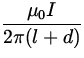=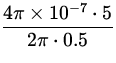= 2.0 x 10- 6 T ,

which is directed into the page. Thus, the net magnetic field is 1.0 x 10- 5 - 2.0 x 10- 6 = 8.0 x 10- 6 T directed out of the page.For the arrangement shown in Fig. 1.14, the long straight wire carries a current of I1 = 5 A. This wire is a distance d = 0.1 m away from a rectangular loop of dimensions a = 0.3 m and b = 0.4 m which carries a current I2 = 10 A. Find the net force exerted on the rectangular loop by the long straight wire.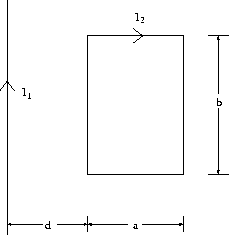Solution:
The force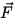= lxon the rectangular loop arises due to the magnetic field B =I/2r of the long straight wire. We first of all note that the force cancels between the top and bottom portions of the loop. Now, on the left portion of the loop,

BL =,

directed into the page, which gives rise to a force

FL = bI2BL === 4 x 10- 5 N ,

which is directed to the left. On the right portion of the loop,

BR =,

directed into the page, which gives rise to a force

FR = bI2BR =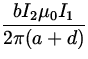=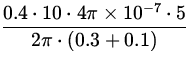= 1 x 10- 5 N ,

which is directed to the right. Thus, the net force is 4 x 10- 5 - 1 x 10- 5 = 3 x 10- 5 N directed to the left.